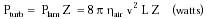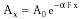Nanomedicine, Volume I: Basic Capabilities

Robert A. Freitas Jr., Nanomedicine, Volume I: Basic Capabilities, Landes Bioscience, Georgetown, TX, 1999

4.9.1.3 Respiratory Audition

The variation of mechanical pressure over a complete respiratory cycle is ~0.003 atm in the pleura, ~0.002 atm at the alveoli, detectable by nanomedical pressure sensors positioned in the vicinity of the respiratory organs. Holding a deep breath further stretches the pulmonary elastic tissue, up to 0.02 atm.

However, turbulent flows at Reynolds number NR > 2300 in the trachea, main bronchus and lobar bronchus produce a whooshing noise that may be the loudest noncardiac sound in the human torso during conventional auscultation. The energy dissipation for turbulent flow in a tube is{Eqn. 4.51}

where Plam is the dissipation for laminar (Poiseuillean) flow in a long circular cylindrical tube of length L, v is the mean flow velocity, and turbulence factor Z = 0.005 (NR3/4 - (2300)3/4), a well-known empirical formula.363 For hair = 1.83 x 10-5 kg/m-sec for room-temperature air (20°C), Pturb = 0.87 milliwatts for the trachea (L = 0.12 m, v = 3.93 m/sec and NR = 4350 at 1 liter/sec volume flow; Table 8.7); Pturb = 0.66 milliwatts for the main bronchus (L = 0.167 m, v = 4.27 m/sec and NR = 3210); and Pturb = 0.09 milliwatts for the lobar bronchus (L = 0.186 m, v = 4.62 m/sec and NR = 2390), totalling ~1.6 milliwatts acoustic emission from a ~120 cm3 upper tracheobroncheal volume. This is a power density of 13 watts/m3 corresponding to a pressure of 4 x 10-5 atm assuming a 300 millisecond measurement window at the maximum respiration rate.

The amplitude of an acoustic plane wave propagating through tissue attenuates exponentially with distance due to absorption, scattering and reflection. The amplitude is given approximately by{Eqn. 4.52}

where A0 is the initial wave amplitude in atm, Ax is the amplitude a distance x from the source, and a is the amplitude absorption coefficient. The function F expresses the frequency dependence of the attenuation. For pure liquids, F = Fliq = n2 (Hz2); for example, aliq = 2.5 x 10-14 sec2/m for water at room temperature. However, for soft tissues, F = Ftiss ~ n (Hz).505* Values for atiss (in sec/m) are in Table 4.2; the value for diamond was estimated from the acoustic line discussion in Section 7.2.5.3. Assuming <10 KHz bronchial turbulence noise of initial amplitude A0 = 4 x 10-5 atm, Ax ~ 3.7 x 105 atm through 1 meter of typical soft tissue; Ax ~ 3.4 x 10-5 atm even if 0.1 meter of bone is interposed in the acoustic path. Either Ax is reliably detected from anywhere in the body using a >(210 nm)3 pressure sensor (Eqn 4.29).

* Most medical ultrasound textbooks assume a ~n dependency of attenuation in soft tissues; apparently, the actual dependency is ~n1.1.730

Last updated on 17 February 2003